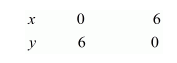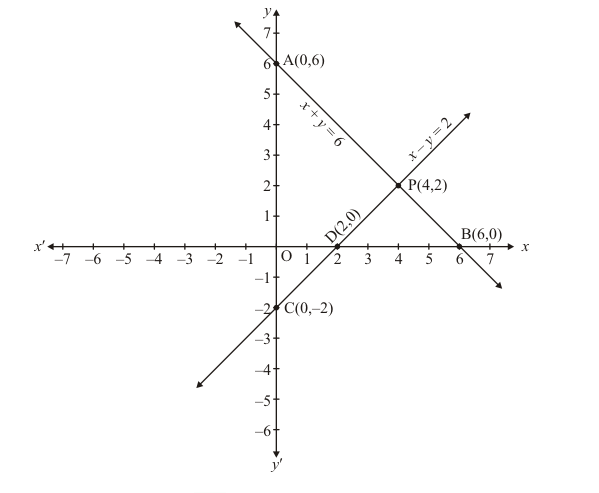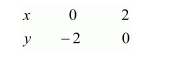# Solve the following systems of equations graphically:`
Question:

Solve the following systems of equations graphically:

$x+y=6$

$x-y=2$

Solution:

The given equations are

$x+y=6 \quad \ldots \ldots .(i)$

$x-y=2 \quad \ldots \ldots \ldots(i i)$

Putting $x=0$ in equation $(i)$, we get:

$\Rightarrow x+0=6$

$\Rightarrow x=6$

$x=0, \quad y=6$

Putting $y=0$ in equation $(i,$, we get:

$\Rightarrow x+0=6$

$\Rightarrow x=6$

$x=6, \quad y=0$

Use the following table to draw the graph.Draw the graph by plotting the two points $A(0,6)$ and $B(6,0)$ from table.Graph of the equation. (ii):

$x-y=2$ ...(ii)

Putting $x=0$ in equation (ii) we get:

$\Rightarrow 0-y=2$

$\Rightarrow y=-2$

$x=0, \quad y=-2$

Putting $y=0$ in equation $($ ii $)$, we get:

$\Rightarrow x-0=2$

$\Rightarrow x=2$

$x=2, \quad y=0$

Use the following table to draw the graph.Draw the graph by plotting the two points $C(0,-2)$ and $D(2,0)$ from table.

The two lines intersect at points $P(4,2)$.

Hence $x=4, \quad y=2$ is the solution.